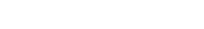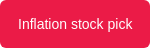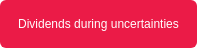## Understanding Earnings-Per-Share (EPS), Price-to-Earnings (P/E) Ratio/

Understanding Earnings per share and P/E Ratio

When investing in the stock market, as companies make profits, investors can use two metrics, earnings per share (EPS) and price-to-earnings (P/E) ratio, to determine how much profit a company generated per share, and whether the share is considered overvalued or undervalued.

Calculating Earnings per Share (EPS)

Investors use the given data from the financial statement to calculate EPS, which reflects how much a company is making per share.

Take for example.

• Company A has net profits of R500 000

With an average of 10 000 outstanding shares.

• Company B has net profits of R700 000

With an average of 40 000 outstanding shares.

1. Company A's EPS will be R500 000/10 000 = R50 per share.
2. Company B's EPS will be R700 000/40 000 = R17.50 per share.

When a company pays dividends, this value is subtracted from the profits before dividing by outstanding shares. This may affect the EPS the same as when a company issues additional shares, reducing the earnings per share.

Calculating price-to-earnings (P/E)

Using the company's EPS and trading price, investors can determine the price-to-earnings (P/E) ratio. This ratio gives investors an idea of whether a stock is overvalued or undervalued (paying more or less than the EPS). This means that investors may be paying too much or too little compared to the earnings.

Example:

Since we've calculated the EPS of company A and B, which is R50 per share and R17.50 per share, assuming the companies are trading at R100 and R60 per share, respectively.

Calculating P/E Ratio

Company A = share price / EPS

• R100 / R50 = 2 x (P/E ratio)

Company B =share price / EPS

• R60 / R17.50 = 3.4 x (P/E ratio)

This means for company A and B, investors are paying 2 times and 3.4 times for the shares of the companies relative to the earnings.

View example using the Inflation stock pick here or belowIn some cases, higher earnings are considered good. At the same time, low earnings may also reflect that the company may be in a development phase - this applies equally to a low or high P/E ratio. A company's earnings are some of the metrics that can reflect the company's profitability within the period. These earnings can be directed to different purposes within the business, including rewards such as bonus shares, share buybacks or dividend pay-outs.

Read Dividends during uncertainties here or belowDividend Payout Ratio

Relating to dividends, earnings per share (EPS) can be used to calculate the dividend pay-out ratio within the period.This refers to the value of funds that are paid out to investors compared to what the company has made per share (earnings per share). Earnings that are not paid out to investors are retained earnings, unless these funds are redirected for another purpose.